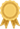### DeltaStock - Global Forex and CFD Broker

Open a Live Account
Open a FREE Demo AccountИнвестиционен посредник в България с най-висок рейтинг за 2017 г.Инвестиционен посредник в България с най-висок рейтинг за 2017 г.
 Lesson

# Interest in trading CFDs on margin

Interest for trading CFDs on margin is an additional amount that is owed when using borrowed funds for the rollover of a position to the next business day and is calculated as a percentage of it. The amount can be a positive or a negative number.

If you have an open position in CFDs on Shares, Indices, ETFs or Cryptocurrencies at the end of the business day, which has to be transferred to the next day, interest is debited (withheld) from or credited (added) to your account.

Note: When trading CFDs on 100% margin (Cash CFDs), interest is neither credited nor debited!

The interest rate determining the interest you will pay or receive for holding an open position is equal to:

• the prime interest rate for the currency in which the respective CFD’s underlying instrument is traded;
• the amount of the increment (positive or negative) over the prime interest rate: depends on whether your position is long or short.

Note: The interest rate may be a positive or a negative number. The amount of interest accrued is calculated, based on the entire value of the position (number of CFDs x closing price) at the end of the business day. Detailed information can be found in the Tariff of Interest Rates, Fees and Commissions of Deltastock.

Interest is calculated using the following formula:

 Interest (daily) = Number of CFDs1 × Closing price × Interest rate 360 days

1 When calculating the interest, the number of CFDs corresponding to the position at the end of the business day is used.

If you have a long position, interest will be withheld from your account.

If you have a short position, interest will be paid to your account when the interest rate on your position is a positive number. Interest will be withheld if the interest rate on your position is a negative number.

Interest is credited or debited in the currency of the traded instrument. If your account is in another currency, then the interest amount will be revaluated (converted) to the currency of the account, using the corresponding closing price for the respective currency pair.

Examples:

Please be advised that prices and interest rates displayed below are for reference purposes only and do not reflect the current market situation.

1) You have a LONG position in CFDs on a certain index:

– Your position is 5 CFDs

– Closing price is 6613.10 EUR

0.75% base interest rate plus 3.0% increment for long positions equals to 3.75% interest rate on the position

5 CFDs × 6613.10 EUR × (0.75 + 3.00) / (100*360) = 3.44 EUR

The interest, in the amount of 3.44 EUR, will be withheld from your account, because the position is long.

2) You have a SHORT position in CFDs on a certain index:

– Your position is -5 CFDs

– Closing price is: 6613.10 EUR

0.75% base interest rate minus 3.0% increment for short positions equals to -2.25% interest on the position

-5 CFDs × 6613.10 EUR × (0.75 – 3.00) / (100*360) = 2.07 EUR

The interest, in the amount of 2.07 EUR, will be withheld from your account, because the position is short, but the interest rate on the position is a negative number (-2.25).

3) You have a SHORT position in CFDs on a certain index:

– Your position is -5 CFDs

– Closing price is 6613.10 EUR

– Let us assume that the base interest rate has risen and is now 3.75% The increment for short positions is minus 3.0%. Therefore, the interest rate on the position equals to 0.75%.

-5 CFDs × 6613.10 EUR × (3.75 – 3.00) / (100*360) = -0.69 EUR

The interest, in the amount of 0.69 EUR, will be added to your account, because the position is short and the interest rate is a positive number (0.75).

4) You have a SHORT position in CFDs on a certain index:

– Your position is -5 CFDs

– Closing price is 6613.10 EUR

– The base interest rate is 0.75%, but Deltastock reduces its increment for short positions down to 0.5%. In this case, 0.75% minus 0.5% equals to 0.25% interest rate on the position

-5 CFDs × 6613.10 EUR × (0.75 - 0.50) / (100*360) = -0.23 EUR

The interest, in the amount of -0.23 EUR, will be added to your account, since because the position is short and the interest rate is a positive number (0.25).

Note: The information displayed on this page is for educational purposes only and is not a personal recommendation or investment advice. Any quotes of financial instruments in the examples are for reference purposes only and do not reflect the current market situation.